# 排队论算法汇总含matlab代码_数学建模(十二)

1.输入过程

(1)组成总体(称为顾客源)的个体(顾客)数可能是有限的，也可能是无限的。
(2)顾客到达系统的方式可能是逐个到达，也可能是成批到达。
(3)前后两个顾客达到系统的时间间隔般是随机的，但也有确定的情形。
(4)顾客到达一般是相互独立，没有互相关联的。但也有关联的情形。
(5)考虑顾客相继达到系统的时间间隔是随机的情形，这时，到达的时间间隔具有一定的概率分布，若该分布中涉及的数字特征，如数学期望、方差等与时间无关，则称为输入过程是平稳的，否则称为非平稳的。
2.排队规则

(1)先到先服务(简记为FCFS)。即按照顾客到达系统的先后次序等待接受服务。在日常生活中，这是最常见的情况。
(2)后到先服务(简记为LCFS)。即后到者先接受服务。比如，仓库中存放同样的原材料，后到者意味着放在最上面或最外面，那么取出该材料即为后到先服务。
(3)随机服务。即服务台或服务员从等待接受服务的顾客中随机选取进行服务。了
(4)有优先权的服务。即按照某种优先权对达到的顾客进行服务。
3.服务机构

X/Y/Z
1971年将Kendall记号扩充为
X/Y/Z/A/B/C

(1)队长。队长是排队系统中的顾客数，这是一个随机变量，其数学期望记为Ls.系统中的顾客数由正在接受服务的顾客数和等待接受服务的顾客数组成。这两者也是随机变量，记等待接受服务的顾客数的数学期望为Lq。一般来说Ls或Lq越大，说明排队系统的服务效率越低，因为这往往意味着顾客需要等待较长的时间才能得到服务。
(2)逗留时间和等待时间。逗留时间指的是顾客在系统中的停留时间，其数学期望记为Ws,等待时间的数学期望记为Wq.显然，逗留时间等于等待时间和接受服务的时间之和。
(3)忙期和闲期。忙期指的是从顾客到达系统开始到顾客离开系统，系统里没有顾客为止的时间段;闲期这是系统里没有顾客到来的时间段。这两个指标反映了系统里服务员的工作强度。一个合理的排队系统不仅关心顾客的等待队长、等待时间等，同时也要关心服务员的工作强度。
(4)损失率。在即时制或排队有限制的系统中，由于正在服务而拒绝后到的顾客或顾客离去使得企业受到损失的概率。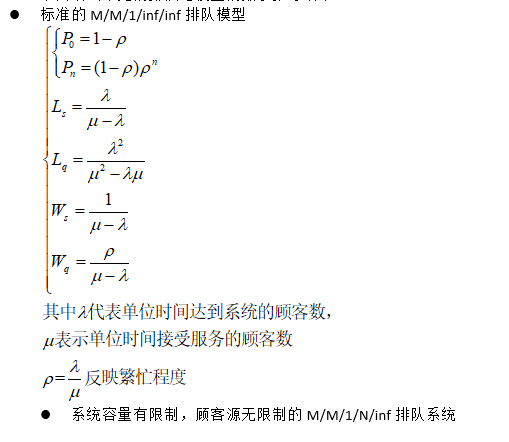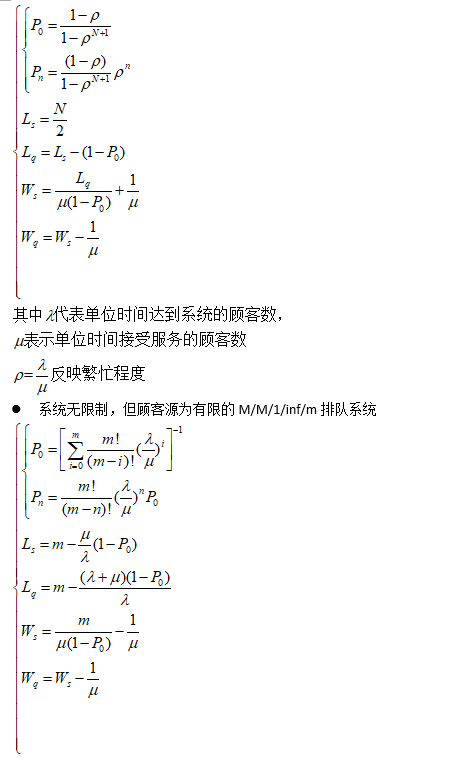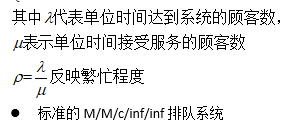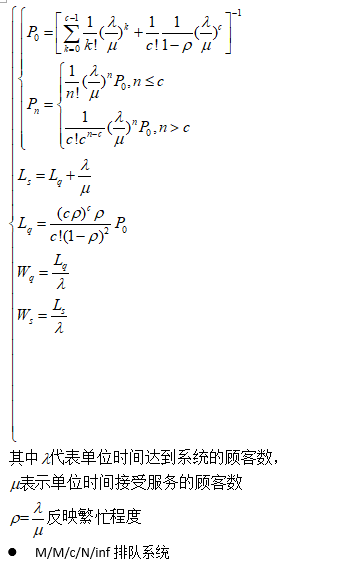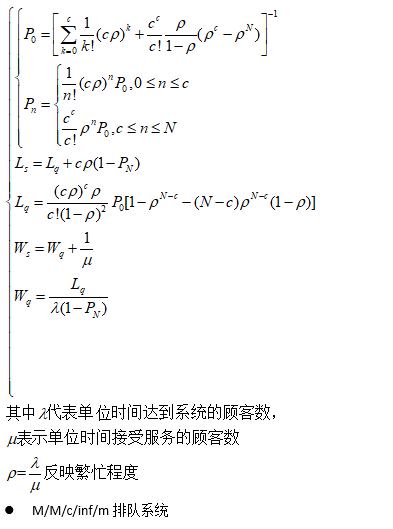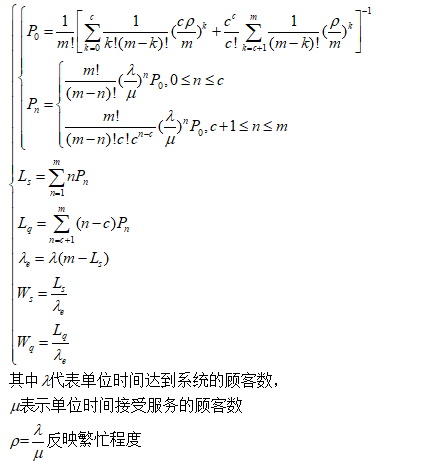Matlab代码：

function [P0,P,Ls,Lq,Ws,Wq] = MMC2(lambda,mu,c,N)
%M/M/c/N/inf排队系统
%lambda为顾客平均达到率
%mu为系统平均服务率
%c为服务器台数
%N为系统中顾客总数的限制
%P0为系统空闲率
%系统中有n个顾客的概率为向量P的第n个分量
%Ls为系统中平均顾客数（平均队长）
%Lq为系统中平均等待接收服务的顾客数（平均等待队长）
%Ws为顾客在系统中的平均逗留时间
%Wq为顾客在系统中的平均等待时间
rho=lambda/(c*mu);
T1=0;
P=zeros(1,N);
for k=0:c
T1=T1+(c*rho)^k/factorial(k);
end
T2=(c^c/factorial(c))*(rho*(rho^c-rho^N)/(1-rho));
P0=(T1+T2)^(-1);
for i=1:c
P(i)=((c*rho)^i/factorial(i))*P0;
end
for i=c:N
P(i)=(c^c/factorial(c))*rho^i*P0;
end
Lq=((P0*rho*(c*rho)^c)/(factorial(c)*(1-rho)^2))*(1-rho^(N-c)-(N-c)*rho^(N-c)*(1-rho));
Ls=Lq+c*rho*(1-P(N));
Wq=Lq/(lambda*(1-P(N)));
Ws=Wq+1/mu;
end

function [P0,P,Ls,Lq,Ws,Wq] = MMC3(lambda,mu,c,m)
%M/M/c/inf/m排队系统
%lambda为顾客平均达到率
%mu为系统平均服务率
%c为服务器台数
%N为系统中顾客总数的限制
%P0为系统空闲率
%系统中有n个顾客的概率为向量P的第n个分量
%Ls为系统中平均顾客数（平均队长）
%Lq为系统中平均等待接收服务的顾客数（平均等待队长）
%Ws为顾客在系统中的平均逗留时间
%Wq为顾客在系统中的平均等待时间
rho=m*lambda/(c*mu);
T1=0;
T2=0;
Ls=0;
Lq=0;
P=zeros(1,m);
for k=0:c
T1=T1+(c*rho/m)^k/(factorial(k)*factorial(m-k));
end
for k=c+1:m
T2=T2+(rho/m)^k/factorial(m-k);
end
P0=(1/factorial(m))*(T1+(c^c/factorial(c))*T2)^(-1);
for i=1:c
P(i)=(factorial(m)/(factorial(m-i)*factorial(i)))*(lambda/mu)^i*P0;
end
for i=c+1:m
P(i)=((factorial(m)/(factorial(m-i)*factorial(c)*c^(i-c))))*(lambda/mu)^i*P0;
end
for k=1:m
Ls=Ls+k*P(k);
end
for k=c+1:m
Lq=Lq+(k-c)*P(k);
end
Le=lambda*(m-Ls);
Wq=Lq/Le;
Ws=Ls/Le;
end



[P0,P,Ls,Lq,Ws,Wq] = MMC2(6,0.5,8,8)
P0 =3.9633e-05
P = 0.0005 0.0029 0.0114 0.0342 0.0822 0.1644 0.2818 0.4227
Ls = 6.9281
Lq = 0
Ws = 2
Wq = 0
[P0,P,Ls,Lq,Ws,Wq] = MMC3(1,4,2,5)
P0 = 0.3149
P = 0.3937 0.1968 0.0738 0.0185 0.0023
Ls = 1.0941
Lq = 0.1176
Ws =0.2801
Wq =0.0301

李工农.运筹学基础及其MATLAB应用.北京：清华大学出版社,2016

01-3008-295万+
03-03
11-24
01-201万+
07-01
07-033543
09-17154
10-12423
08-251383
01-29
10-23219
04-23
01-231万+
02-05828
04-063341
12-131576
12-051710
05-021万+